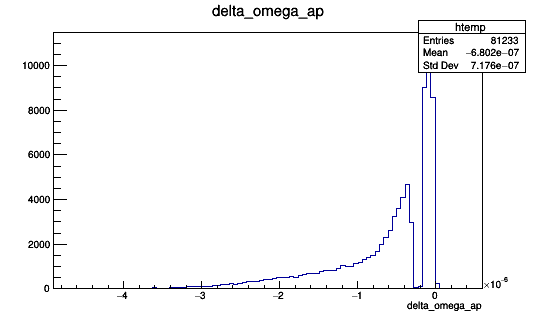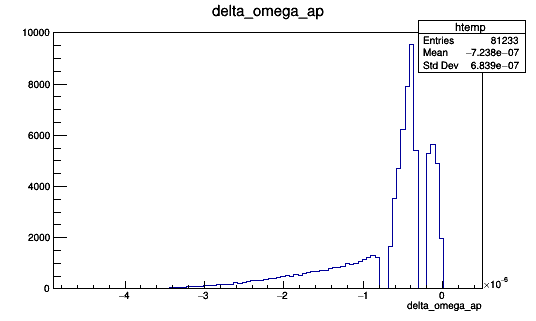# Discontinuity in distribution of fit values

I am plotting a histogram with the results of fitting 81233 TGraph objects.

For the fit I define the function `TF1 *Cosine = new TF1("Cosine", "*cos(*x*1.e6 + )+", 0., tmax)` and then

``````Cosine->SetParameters(A0, omega*1.e-6, phi0, 0.);
Cosine->SetParLimits(0, -1., 1.);
Cosine->SetParLimits(2, 0., M_PI); //acos have a range of 0,pi

gr->Fit("Cosine", "WRM");
``````

The problem is that as you can see in the plot attached, there are almost no values around -0.3. This does not makes sense physically (the distribution should be smooth and continuous), and I checked that the data I am fitting is correct. I suspect that there is a problem when I do the fit, but I can’t think of anything.You fit the “gr” graph and get the “htemp” histogram?

``````{
Double_t x, y;
Int_t n = 20;
for (Int_t i=0;i<n;i++) {
x[i] = i*0.1;
y[i] = 10*sin(x[i]+0.2);
}
gr = new TGraph(n,x,y);

TF1 *Cosine = new TF1("Cosine", " * cos( * x + ) + ",
gr->GetXaxis()->GetXmin(), gr->GetXaxis()->GetXmax());
Cosine->SetParameters(1., 2., 3., 4.);
Cosine->SetParLimits(2, 0., TMath::TwoPi());
gr->Fit("Cosine", "WRM");

gStyle->SetOptFit(112);
gr->Draw("A*");
}
``````

I store the values of the parameter  in a TTree, and the histogram I attached is the histogram produced by the TBrowser when I open that TTree.

I guess you could draw this parameter () versus the chi^2 of the fit, versus another parameters, versus the graph number and see if you find any unexpected / strange “correlations”.

I did what you suggested, but I can´t find any strange correlation. I tried to give the -0.3e-6 as initial value, and as you can see in the plot attached, the gap is displaced now to -0.5e-6.Giving now -0.5e-6 as initial value, the gap is displaced and a new ones appear. I am using the same data all the time. I believe this is a problem of the precision when fitting.You can also try to inspect the: `Int_t fitStatus = gr->Fit("Cosine", "WRM");`
Search for “Access to the fit” (“result” and “status”) in the TGraph::Fit method description.